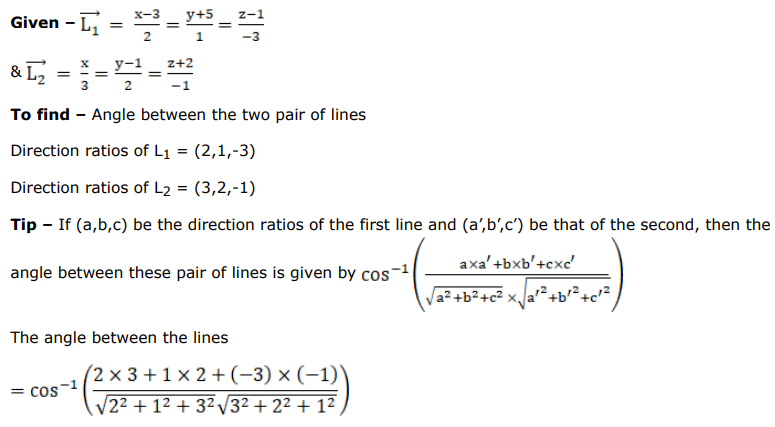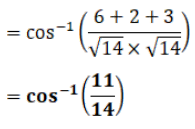# Find the angle between each of the following pairs of lines:Question:

Find the angle between each of the following pairs of lines:

$\frac{3-x}{-2}=\frac{y+5}{1}=\frac{1-z}{3}$ and $\frac{x}{3}=\frac{1-y}{-2}=\frac{z+2}{-1}$

Solution: